## How to Subtract Decimals?

Practice Unlimited Questions

#### How to Subtract Decimals?

Step 1: Align the decimal points.

Step 2: Subtract the Ones.

Step 3: Subtract the Tenths.

See working examples below.

#### 1. Bob has 0.3 kg of butter and Bill has 0.8 kg of butter. How much more butter has Bill than Bob?

Subtract 0.3 and 0.8

0.8  −  0.3  =  ?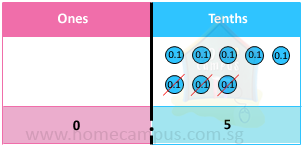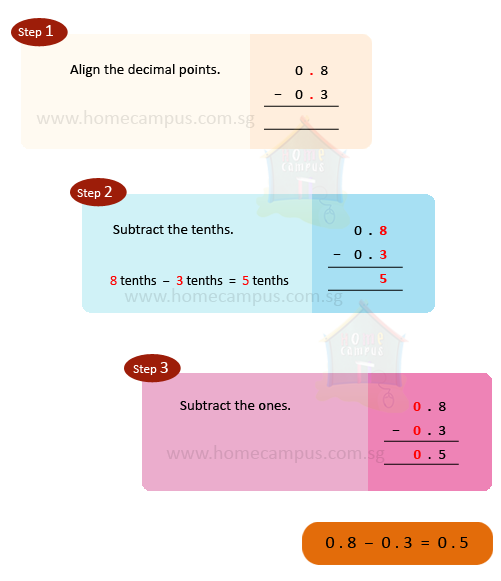Bill has 0.5 kg more butter than Bob.

#### 2. Bob has 0.8 L of milk and Bill has 2.3 L of milk. How much more milk has Bill than Bob?

Subtract 0.8 from 2.3

2.3  −  0.8  =  ?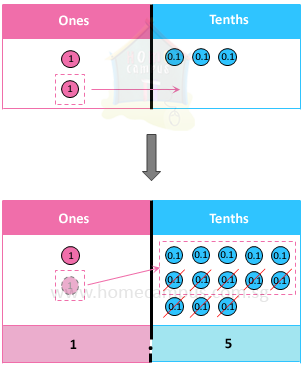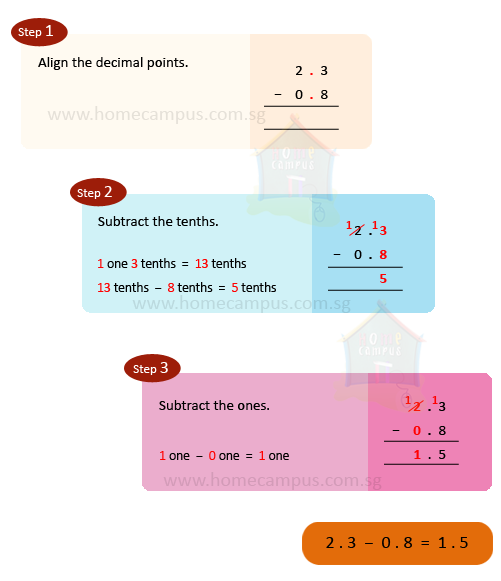Bill has 1.5 L more milk than Bob.

#### 3. Bob has 3.5 kg of flour and Bill has 2.67 kg of flour. How much more flour has Bob than Bill?

Subtract 2.67 from 3.5

3.5  −  2.67  =  ?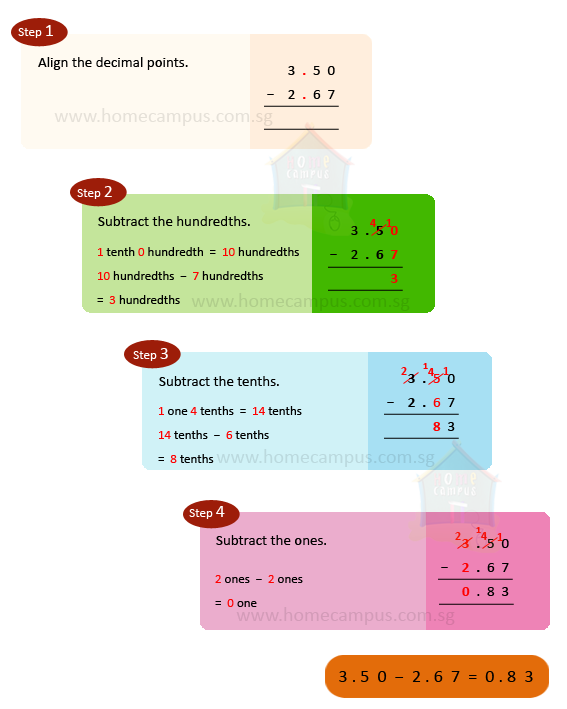Bob has 0.83 kg more flour than Bill.

#### 4. Serena had \$20 at first. How much money had she left after spending \$12.34 at a stationery shop?

Sum of money at first   =   \$20.00
Sum of money spent   =   \$12.34
\$20  =  \$20.00
Sum of money left   =   \$20.00  −  \$12.34
=   \$7.66